# Class 10 NCERT Solutions- Chapter 13 Surface Areas And Volumes – Exercise 13.5

• Difficulty Level : Medium
• Last Updated : 04 Mar, 2021

### Question 1. A copper wire, 3 mm in diameter, is wound about a cylinder whose length is 12 cm, and diameter 10 cm, so as to cover the curved surface of the cylinder. Find the length and mass of the wire, assuming the density of copper to be 8.88 g per cm3.

Solution:

Given,

Radius of copper wire (r) =mm =cm

Height of cylinder (H) = 12 cm

Radius of Cylinder (R) == 5 cm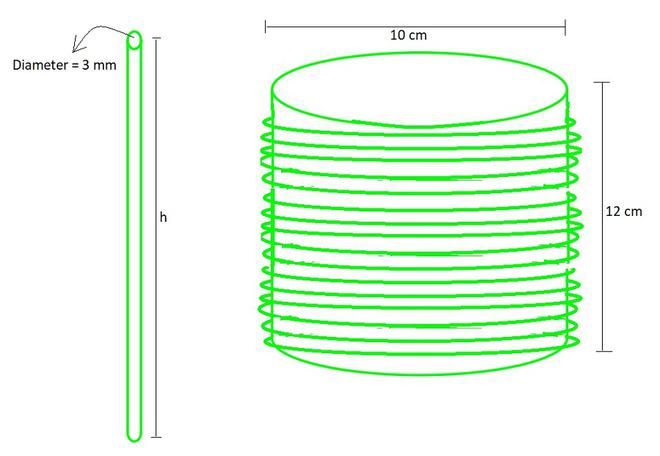Length of copper wire needed for one round will be circumference of cylindrical circle end = 2πR

=2π(5)

length of each round = 10π cm

Now, to completely cover the cylinder with wire no of rounds (n) of copper wire should be:

n = height of cylinder / diameter of copper wire

n =no. of rounds = 40

Now, Total length of copper wire will be:

h = no. of rounds × length of each round

h = 40×10π

h = 400×3.14                            (taking π = 3.14)

h = 1256 cm

Volume of copper wire = πr2h

=π×()2×1256

=π××1256

= 88.82 cm3                                (taking π =)

Now, as it is given that,

For 1cm3 = 8.88 g of wire

so, for 88.82 cm3 = 8.88×88.82 g

Mass of copper wire = 788.7216 g

Hence, the length and mass of the wire are1256 cm and 788.216 grams respectively.

### Question 2. A right triangle, whose sides are 3 cm and 4 cm (other than hypotenuse) is made to revolve about its hypotenuse. Find the volume and surface area of the double cone so formed. (Choose value of π as found appropriate.)

Solution:

After revolving the triangle about its hypotenuse, we get two cones having slant height as 3 cm and 4 cm each.

Hypotenuse of triangle (CD) = √(32 + 42) = 5 cm

l1 = 3 cm

l2 = 4 cm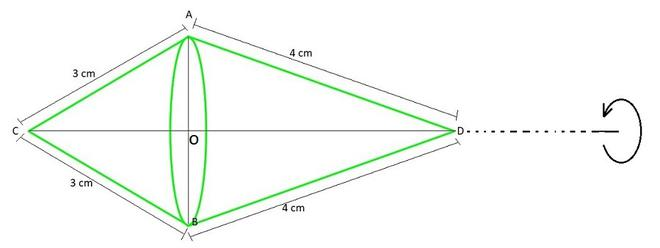ar(△ACD)×AC×AD = ½×AO×CD         (as AC⊥AD and AO⊥CD)

⇒ AC×AD = AO×CD

⇒ 4×3 = AO×5

AO =cm

Hence, radius of cone base iscm

Now, Volume of double cone = Volume of cone 1 + Volume of cone 2

= ()πr2×OD + ()πr2×OC

= ()πr2×(OD+OC)πr2×CD= 30.17 cm3

Now, Curve Surface area of double cone = CSA of cone 1 + CSA of cone 2

= πrl1 + πrl2

= πr(l1+l2)×(3+4)

= 30.17 cm2

Hence, the volume and surface area of the double cone so formed is 30.17 cm3 and 30.17 cm2 .

### Question 3. A cistern, internally measuring 150 cm × 120 cm × 110 cm, has 129600 cm3 of water in it. Porous bricks are placed in the water until the cistern is full to the brim. Each brick absorbs one-seventeenth of its own volume of water. How many bricks can be put in without overflowing the water, each brick being 22.5 cm × 7.5 cm × 6.5 cm?

Solution:

Given,

Length of Cistern (L) = 150 cm

Breadth of Cistern (W) = 120 cm

Height of Cistern (H) = 110 cm

Length of Brick (l) = 22.5 cm

Breadth of Brick (w) = 7.5 cm

Height of Brick (h) = 6.5 cm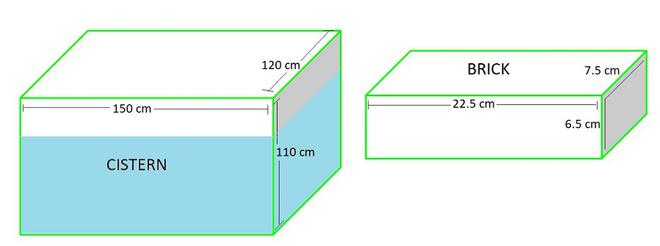Volume of Cistern = L×B×H

= 150×120×110

= 1980000 cm3

Volume of Water =  129600 cm3

Empty space left in Cistern = 1980000-129600

= 1850400 cm3

Volume of Brick = l×b×h

= 22.5×7.5×6.5

= 1096.88 cm3

Volume of n Bricks = 1096.88×n cm3

Volume absorbed by each brick = ()th (volume of brick)×1096.88 cm3

= 64.522 cm3

Then, Volume absorbed by n bricks = 64.522×n

Volume of brick = Empty space left in Cistern + volume absorbed by bricks

1096.88×n = 1850400 + 64.522×n

n×(1096.88-64.522) = 1850400

n =n = 1792.40

n ≈ 1792

Hence, 1792 bricks can be put in without overflowing the water.

### Question 4. In one fortnight of a given month, there was a rainfall of 10 cm in a river valley. If the area of the valley is 7280 km2, show that the total rainfall was approximately equivalent to the addition to the normal water of three rivers each 1072 km long, 75 m wide and 3 m deep.

Solution:

Length of river (l) = 1072 km

Width of river (w) =km = 0.075 km

Depth of river (h) == 0.003 km

Depth of rainfall =km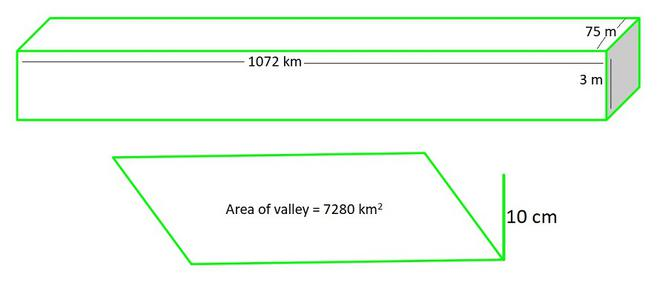The total rainfall was approximately equivalent to the addition to the normal water of three rivers each

Which means,

(Volume of three rivers = ((Surface area of a valley)×Depth of rainfall) has to be equal.

Let’s check, for each case,

so,

Volume of three rivers = 3×(l×b×h)

= 3×(1072×0.075×0.003) km3

= 0.7236 km3 ………………………..(1)

Volume of rainfall in valley = Area of valley×depth of rainfall

= 7280×= 0.728 km3……………………………….(2)

From (1) and (2) we can see that,

Hence, proved, Total rainfall was approximately equivalent to the addition to the normal water of three rivers each.

### Question 5. An oil funnel made of tin sheet consists of a 10 cm long cylindrical portion attached to a frustum of a cone. If the total height is 22 cm, diameter of the cylindrical portion is 8 cm and the diameter of the top of the funnel is 18 cm, find the area of the tin sheet required to make the funnel (see Fig. 13.25).

Solution:

Oil funnel contains two shapes = frustum + Cylinder

Given values,

Larger radius of frustum (R) == 9 cm

Smaller radius of frustum (r) == 4 cm

Height of frustum (H) = 22-10 = 12 cm

Radius of cylinder (r) == 4 cm

Height of cylinder (h) = 10 cm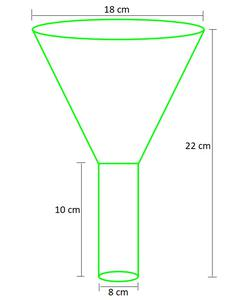Area of the tin sheet required = CSA of frustum + CSA of cylinder

CSA of frustum = π(r+R)l

= π×(9+4)×√(122 + (9-4)2)                       (l =√(H2 + (R-r)2))

= π×13×13

= 169π cm2

CSA of cylinder = 2πrh

= 2×π×4×10

= 80π cm2

Area of the tin sheet required = 169π + 80π

= 249π

= 782.571 cm2                                                    (Taking π=)

Hence, the area of the tin sheet required to make the funnel is 782.571 cm2

### Question 6. Derive the formula for the curved surface area and total surface area of the frustum of a cone, given to you in Section 13.5, using the symbols as explained.

Solution:

Let ADE be a cone. From the cone the frustum BCDE is cut by a plane parallel to its base. Here, r and R are the radii of the frustum ends of the cone and h be the frustum height.

Here, as BC||DE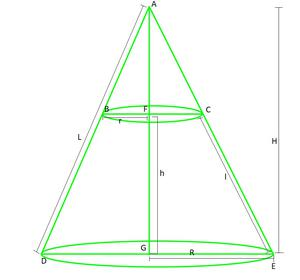In △ADG and △ABF

So, ΔADG ∼ ΔABF (as BC||DE)…………..(1)

from (1), we get

1-() == 1 –=L(R-r) = Rl………………………(2)                 (By rearranging)

Total surface area of frustum = CSA of frustum + Area of upper circular end + Area of the lower circular end

• CSA of frustum = CSA of cone ADE – CSA of cone ABC

= πRL – πr(L-l)

= πL(R-r)+πrl

= πRl+πrl                 (from (2) replacing L(R-r) = Rl )

= π(R+r)l

• Area of upper circular end = πR2
• Area of the lower circular end = πr2

Total surface area of frustum = π(R+r)l + πR2 + πr2

### Question 7. Derive the formula for the volume of the frustum of a cone, given to you in Section 13.5, using the symbols as explained.

Solution:

Let ADE be a cone. From the cone the frustum BCDE is cut by a plane parallel to its base. Here, r and R are the radii of the frustum ends of the cone and h be the frustum height.

Here, as BC||DEIn △ADG and △ABF

So, ΔADG ∼ ΔABF (as BC||DE)…………………(1)

from (1), we get

1-() == 1 –=H(R-r) = Rh………………………(2)                   (By rearranging)

Total volume of frustum of the cone will be = Volume of cone ADE – Volume of cone ABCπR2H –πr2(H-h)πR2H –πr2H +πr2hπ(H(R2-r2)+r2h)π(H(R-r)(R+r)+r2h)                          (Replacing (R2-r2) = (R-r)(R+r))π(Rh(R+r)+r2h)                                (from (2) replacing H(R-r) = Rh )π(R2h+Rrh+r2h)πh(R2+Rr+r2)                      (Taking h common)

Hence, Total volume of frustum of the cone will be =πh(R2+Rr+r2

My Personal Notes arrow_drop_up